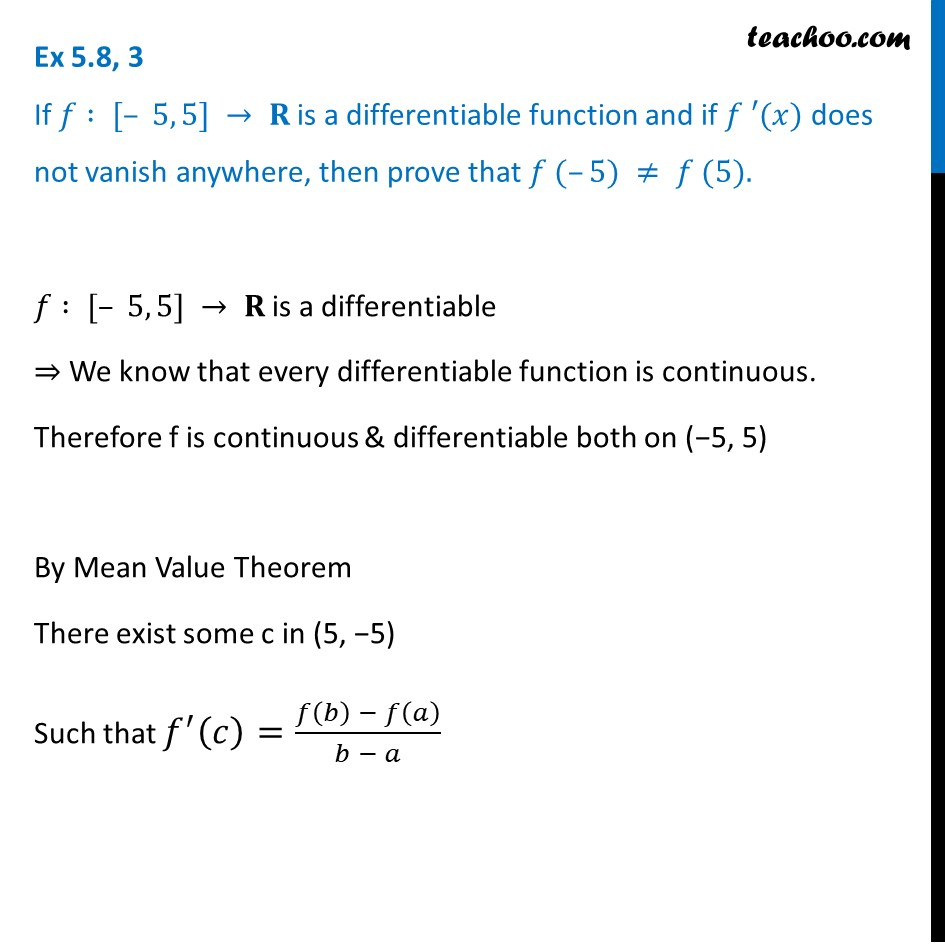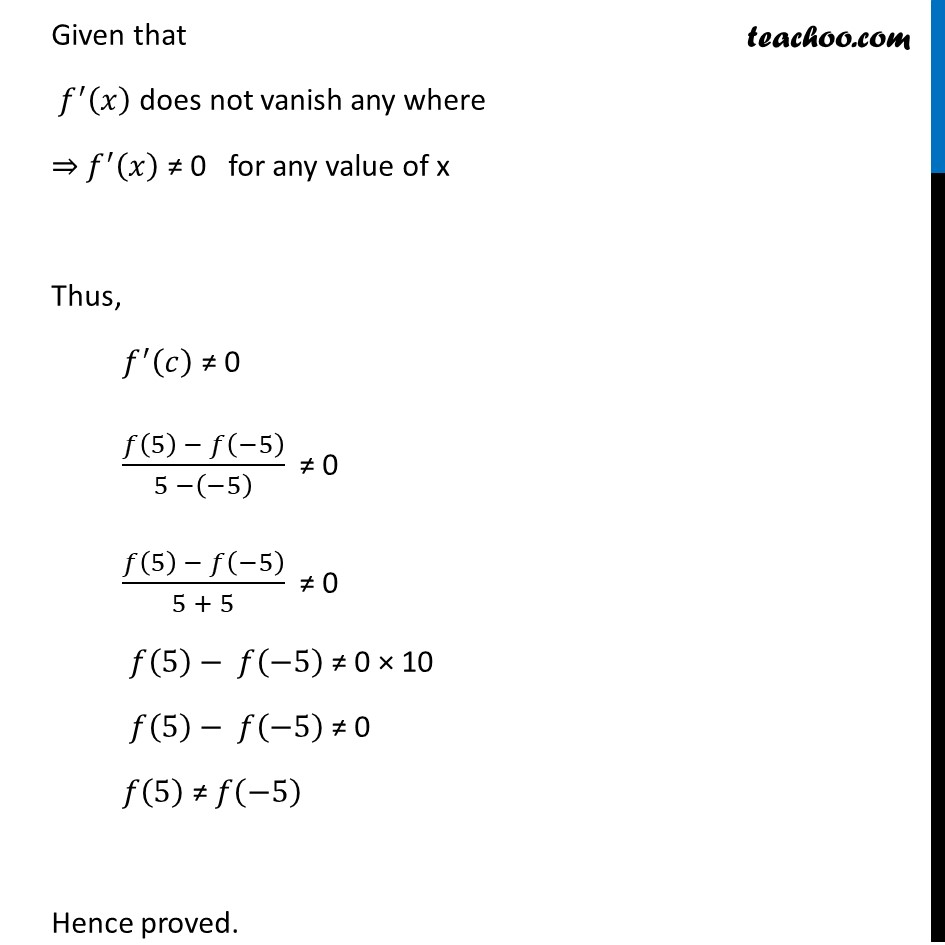Rolle's and Mean Value Theorem

Chapter 5 Class 12 Continuity and Differentiability
Serial order wiseLearn in your speed, with individual attention - Teachoo Maths 1-on-1 Class

### Transcript

Question 3 If 𝑓 : [– 5, 5] → 𝐑 is a differentiable function and if 𝑓 ′(𝑥) does not vanish anywhere, then prove that 𝑓 (–5) ≠ 𝑓 (5). 𝑓 : [– 5, 5] → 𝐑 is a differentiable ⇒ We know that every differentiable function is continuous. Therefore f is continuous & differentiable both on (−5, 5) By Mean Value Theorem There exist some c in (5, −5) Such that 𝑓^′ (𝑐)=(𝑓(𝑏) − 𝑓(𝑎))/(𝑏 − 𝑎) Given that 𝑓^′ (𝑥) does not vanish any where ⇒ 𝑓^′ (𝑥) ≠ 0 for any value of x Thus, 𝑓^′ (𝑐) ≠ 0 (𝑓(5) − 𝑓(−5))/(5 −(−5) ) ≠ 0 (𝑓(5) − 𝑓(−5))/(5 + 5) ≠ 0 𝑓(5)− 𝑓(−5) ≠ 0 × 10 𝑓(5)− 𝑓(−5) ≠ 0 𝑓(5) "≠" 𝑓(−5) Hence proved.